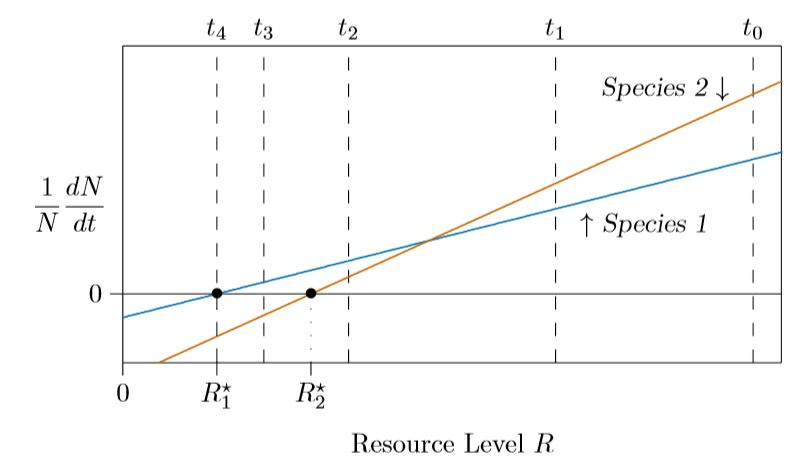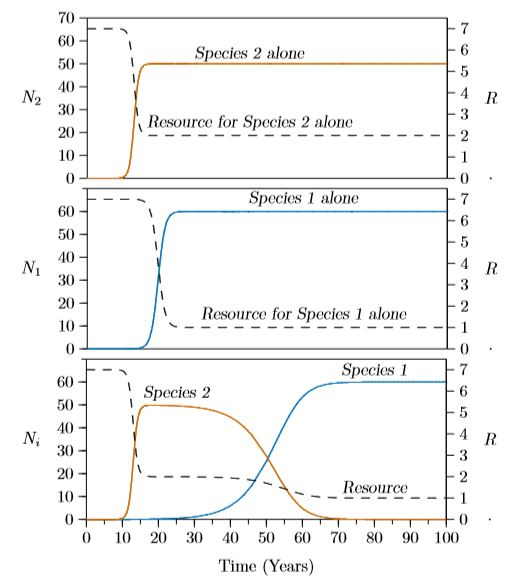# 16.4: Competitive exclusion

$$\newcommand{\vecs}{\overset { \rightharpoonup} {\mathbf{#1}} }$$ $$\newcommand{\vecd}{\overset{-\!-\!\rightharpoonup}{\vphantom{a}\smash {#1}}}$$$$\newcommand{\id}{\mathrm{id}}$$ $$\newcommand{\Span}{\mathrm{span}}$$ $$\newcommand{\kernel}{\mathrm{null}\,}$$ $$\newcommand{\range}{\mathrm{range}\,}$$ $$\newcommand{\RealPart}{\mathrm{Re}}$$ $$\newcommand{\ImaginaryPart}{\mathrm{Im}}$$ $$\newcommand{\Argument}{\mathrm{Arg}}$$ $$\newcommand{\norm}{\| #1 \|}$$ $$\newcommand{\inner}{\langle #1, #2 \rangle}$$ $$\newcommand{\Span}{\mathrm{span}}$$ $$\newcommand{\id}{\mathrm{id}}$$ $$\newcommand{\Span}{\mathrm{span}}$$ $$\newcommand{\kernel}{\mathrm{null}\,}$$ $$\newcommand{\range}{\mathrm{range}\,}$$ $$\newcommand{\RealPart}{\mathrm{Re}}$$ $$\newcommand{\ImaginaryPart}{\mathrm{Im}}$$ $$\newcommand{\Argument}{\mathrm{Arg}}$$ $$\newcommand{\norm}{\| #1 \|}$$ $$\newcommand{\inner}{\langle #1, #2 \rangle}$$ $$\newcommand{\Span}{\mathrm{span}}$$

Consider what will happen with two species using the same resource, such as light or space or nitrogen fertilizer. The amount of resource available will be the maximum amount, $$R_{max}$$, minus what is tied up in all individuals of all species. With $$u_i$$ being the amount of resource tied up in each individual of species $$i$$, the resource remaining at any time will be

$$R\,=\,R_{max}\,-\,u_1N_1\,-\,u_2N_2$$

Or for many species

$$R\,=\,R_{max}\,-\,u_1N_1\,-\,u_2N_2\,-u_3N_3\,-\cdots\,-u_hN_h$$

$R\,=\,R_{max}\,-\sum_{i=1}^h u_iN_i$

Each species has its own growth equation, identical in form for all species, but different in the critical level of resource, $$R_i^{\ast}$$, and the growth coefficient, $$m_i$$:

$\frac{1}{N_i}\frac{dN_i}{dt}\,=\,m_i(R\,-\,R_i^{\ast})$

What remains is to consider how the growth coefficient $$m_i$$ relates to the minimal level of resource tolerated, $$R_i^{\ast}$$. It turns out to be a tradeoff between the two. Consider, for example, a plant species that is limited by the amount of nitrogen available, as plants are. And to have a large growth coefficient $$m_i$$ the plant must produce abundant seed. To have superior nitrogen use, measured by a low value of $$R^{\ast}$$, it needs abundant roots. But it cannot do both. There is a limited amount of solar energy to exploit, so if the plant allocates more to roots there is less to allocate to seeds, and vice versa.Figure $$\PageIndex{1}$$. Tradeoff measured between colonization and ability to exploit nitrogen (Tilman 1994, Ecology 75:2–16).

It therefore turns out that species which are good colonizers, producing abundant seed, are poorer competitors for resources, having a higher value of $$R^{\ast}$$. This idea is illustrated by measurements reported in Figure $$\PageIndex{1}$$.Figure $$\PageIndex{2}$$. Two species individual growth rate versus resource level, whose time-course is illustrated in Figure $$\PageIndex{1}$$

In Figure $$\PageIndex{2}$$, tradeoffs are formulated for modeling. Species 2 grows more rapidly when resources are abundant. This is the case at time 0, marked with $$t_0$$ on the top axis.

As the populations grow they reduce the amount of resource available in the environment. At time 1, marked with $$t_1$$ on the upper axis, Species 2 can continue to grow faster than Species 1, though the margin is deteriorating. But there comes a point at which the resources become depleted enough that the characteristics of Species 2 do not let it gather enough resources to maintain its advantage. This is the crossing point of the blue and red lines in the figure. At time 2, both species are still growing, but Species 1 is growing faster. At time 3, with still lower resource levels—drawn down by Species 1—the resource falls below the minimal level for Species 2, $$R_2^{\ast}$$. The growth rate of Species 2 falls negative and Species 2 starts to die out.

Finally, at time 4, Species 1 depletes the resource to the level that it can just barely survive, and it stands alone, having wiped out its competitor. This process is called “competitive exclusion.”

How this plays out over time is illustrated in Figure $$\PageIndex{3}$$. At the top, Species 2 alone does just fine, rapidly rising to its carrying capacity of 50 and pulling the resource down to its $$R^{\ast}\ ) of 2. In the middle, Species 1 alone also does just fine, rapidly rising to its carrying capacity of 60 and pulling the resource down to its \(R^{\ast}$$ of 1.

But grown together, Species 2 makes an initial splash and then declines. This is due to the incessant growth of Species 1, which outcompetes it. Species 1 simply draws the resource down below the level at which Species 2 can survive.

Competitive exclusion, which assumed that no more species could exist than there were resources, was treated as an inviolable law of ecology for over fifty years. In the 1970s, however, this was shown not to be the case (Armstrong and McGehee 1980). More about that later in the chapter.Figure $$\PageIndex{3}$$. Competitive exclusion based on the tradeoffs of Figure $$\PageIndex{2}$$.

This page titled 16.4: Competitive exclusion is shared under a CC BY-NC 4.0 license and was authored, remixed, and/or curated by Clarence Lehman, Shelby Loberg, & Adam Clark (University of Minnesota Libraries Publishing) via source content that was edited to the style and standards of the LibreTexts platform; a detailed edit history is available upon request.